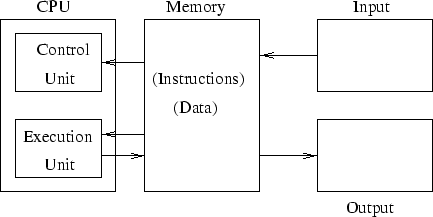# Computers, Software, and Data

Tom Kelliher, CS 102

Sept. 1, 2006

### Announcements

First quiz in one week. See sample quiz.

### Assignment

Be able to answer these questions from pp. 40-42: 12, 21, 25, 28, and 31.

### From Last Time

Syllabus, survey.

### Outline

1. Computers, Software, and Data.

### Coming Up

Inside a computer.

## What's a Computer?

A block diagram:1. What is memory? How does it differ from storage?

2. What parts of a PC are considered input devices? Output devices? Both?

## How is Software Run?

Consider the following simple program:

 1:   let sum = 0
2:   print "How many numbers? "
4:   let loopCount = count
5:   if loopCount equals 0 goto 11
6:   print "Next number: "
8:   let sum = sum + input
9:   let loopCount = loopCount - 1
10:   goto 5
11:   let average = sum / count
12:   print "The average is:", average, "."
13:   end

1. Where do we begin?

2. After completing one step, where do we proceed?

3. Operations: assignment, arithmetic, decision, branch, I/O.

4. Operands: Variables, constants (numeric and string).

## How is Data Kept?

1. Computers use the binary system. Why?

2. Binary digits.

Conversion between binary and decimal is fairly simple, but tedious -- write/use a program.

3. Bits, bytes, words.

4. Memory locations: cells with addresses.

5. How do we represent characters? ASCII code:
1. A: 01000001

2. 4: 00110100

6. How does the computer know if a memory location contains numbers, characters, variables, or instructions?

Thomas P. Kelliher 2006-08-30
Tom Kelliher